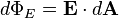Home » Ask Physics » Electric Flux Misconception

Electric Flux Misconception

“Could you please tell me if electric flux is defined as no of lines passing through the area then its ans should only be scalar like 5,8,1,6 etc. why its units are given?”

[warning]Electric Flux is a scalar quantity[/warning]

Please note that electric field lines are imaginary lines to represent electric field diagrammatically. And the definition of electric flux in terms of electric lines of force is just a visual aid to understand the concept of electric flux. The actual definition is not in terms of electric lines of force. If it were so, try finding the answers to these questions too and you will realise the problem

1. “If electric flux through a spherical surface around a point charge is one, then you may feel that there is only one line of force. In which direction will it be? By symmetry, there must  be electric field in all directions around a point charge”
2. “What is the electric flux due to the smallest charge existing freely, i.e; the charge of an electron?  When calculated, it comes to be of the order of 2 x 10-8. How can the number of lines be a fraction?

Please see the definintion of electric flux here:

Wikipedia:

“The electric fluxthrough a small areais given by(the electric field, E, multiplied by the component of area perpendicular to the field). The electric flux over a surface S is therefore given by the surface integral:where E is the electric field and dA is a differential area on the closed surface S with an outward facing surface normal defining its direction.”

Hyperphysics:

“The electric flux through a planar area is defined as the electric field times the component of the area perpendicular to the field.”

Watch a video on electric flux here

Visitors So Far @ AskPhysics

• 2,155,753 hits

Subscribe to Blog via EmailAsk Physics